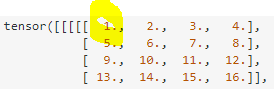# Extraction patches cnn

when I get patches, how do I get the (x, y) axes of the pixel ?

``````pixel=image.reshape(image.shape, image.shape*image.shape)
``````

how do I know the axes of the pixel (x, y) plz

if `c, h, w = image.shape` and the index into the `pixel` tensor is `i, j`, then `y = j // w`, `x = j % w`.

Best regards

Thomas

@tom how do I know the i and j of the pixel?

The instruction you posted “flattens” the image, so you transform the x y index into a a single j index. You can then use any j between 0 and h * w - 1.

@tom , I made a patch extraction in an original image but I want to know i and j of the pixel 1 ?